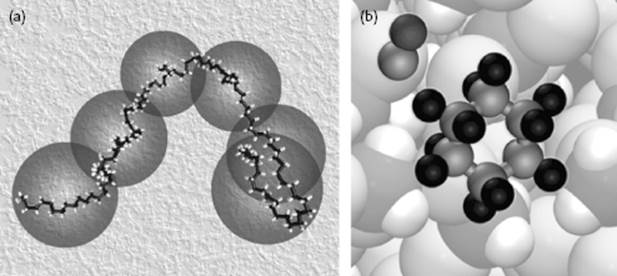﻿ ﻿Outline and Approach - Introduction - Liquid-State Physical Chemistry: Fundamentals, Modeling, and Applications (2013)

## Liquid-State Physical Chemistry: Fundamentals, Modeling, and Applications (2013)

### 1.3. Outline and Approach

From these brief descriptions of liquids and their applications, it will be clear that not all topics can be treated within reasonable limits, even at an introductory level. Hence, it has been decided to discuss those liquids and those properties that are the most relevant to chemical engineers and chemists in average daily practice. Consequently, attention will be limited to classical molecular liquids and solutions, which means that quantum fluids, molten metals, molten salts and highly concentrated ionic solutions are not dealt with. Neither are liquid crystals discussed, since that topic has been treated recently in two introductory books . Here, the focus is on structure and thermodynamic aspects such as activity coefficients and phase behavior. Although some of the topics dealt with are also covered in books on physical chemistry and statistical mechanics, it was felt that a coherent presentation for the audience indicated was missing. Generally, we refrain from discussing experimental methods and focus on the underlying theoretical concepts.

Problems provide an essential part of this text, and the reader is encouraged to study these. Some of the text and some of the problems are somewhat more advanced compared to the remainder, but they are not essential to the core of this book. These sections and problems have been indicated with asterisks.

In order to be sufficiently self-contained, the book starts with the basic aspects of thermodynamics, classical mechanics and quantum mechanics (Chapter 2), after which intermolecular interactions (Chapter 3) and the phenomenology of liquids (Chapter 4) are briefly described. Thus, these chapters review the basics required for the remainder of these notes. Basic to the solution of all problems in liquids is the use of statistical thermodynamics, as discussed in Chapter 5. Subsequently, the meaning of the “structure” and “energetics” of liquids are discussed in Chapter 6. Chapters 5 and 6 largely provide the framework in which the behavior of liquids and solutions is discussed, while the various approaches to simple liquids are discussed in Chapters 7, 8, and 9. An approach that, at least in principle, is rigorous is the integral equation approach (Chapter 7) of which a sketch is provided. However, a solution to a problem in principle is not always a solution in practice, and since this is the case for the integral equation approach, other methods are called for. Physical models (Chapter 8) have been developed in the past and can still be used to advantage for basic understanding. In the present era, computers form an essential tool for scientists, and consequently modern numerical methods such as molecular dynamics and Monte Carlo simulations (Chapter 9) are outlined. Chapters 7, 8 and 9 provide the most frequently encountered approaches towards pure liquids. Polar liquids, for which dipole moment and polarizability play a dominant role, comprise the next level of complexity (Chapter 10). Water is an important example which is discussed somewhat extensively (Figure 1.4). Thereafter, the discussions relate to solutions – that is, liquid molecular mixtures. As a useful guide for mixtures, the regular solution concept is used. This concept has a long history, dating back to J.J. van Laar  (1860–1938), and is particularly useful because of its conceptual simplicity, widespread use, and inherent possibilities for systematic improvement. Solutions with a comparable size of solvent and solute, and those with a rather different size of solvent and solute, can be distinguished. The first category can be subdivided in molecular (nonelectrolyte) solutions, which are treated in Chapter 11, and electrolyte solutions which are discussed in Chapter 12. In Chapter 11 the compatibility of solvents, including phase separation, forms an important part. The dissolution of salts, the structure of water around ions and the calculation of activity coefficients of ions in solution comprise a large part of Chapter 12 (Figure 1.5). The second category contains polymeric solutions and is treated in Chapter 13. The essential differences with molecular solutions and their consequences are treated (Figure 1.6).

Figure 1.4 Water and its structure. (a) A snapshot of a molecular dynamics simulation of water; (b) The coordination of water molecules, indicating the hydrogen bonds.Figure 1.5 Ionic solutions. (a) Dissolution of salts by solvation; (b) The configuration of water molecules around an ion.Figure 1.6 (a) Polymeric solution showing one real chain and its coarse-grained representation in a background of solvent (not shown); (b) Schematic of a reaction between cyclohexane and CN.Some specials topics are briefly introduced in the last three chapters. First, because most chemical reactions are carried out in liquids, in Chapter 14 we turn to the physical influence of solvents on chemical reactions (Figure 1.6). Second, we deal with surfaces (Chapter 15) in view of the relatively large importance of surfaces in fluids. Finally, phase transitions (Chapter 16) are treated as an example of an intimate mixture between molecular and mesoscopic arguments. The Appendices deal with physical constants, some useful mathematics, a brief review of the elements of electrostatics, the lattice gas model, some physical property data, and numerical answers to selected problems.

As with all fields of science and technology, there is an extensive literature available. In the section “Further Reading” a list of books is provided that can be selected for further study. Each chapter also contains, apart from specific references given as footnotes, a section “Further Reading” referring to small set of books relevant to that chapter. When used in the text, these are referred to by “author (year)” or “(author, year)”.

﻿